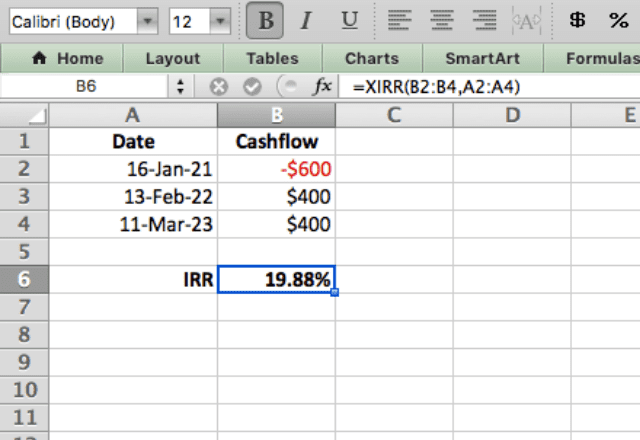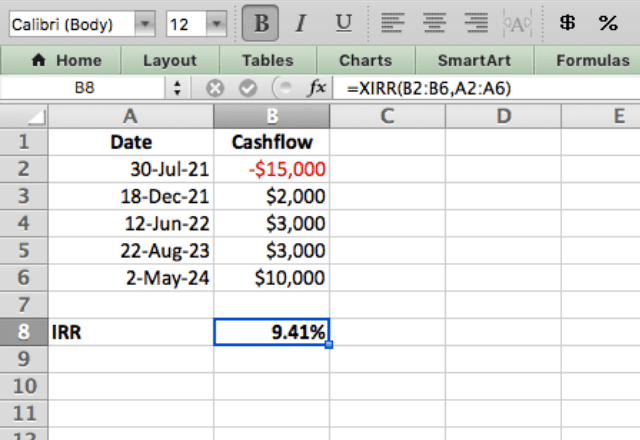# How to use the XIRR function in ExcelThe XIRR function in Excel calculates the internal rate of return, or IRR, of a series of cash flows. The function is designed specifically to handle irregular cash flows that don’t follow a consistent time period.

This makes the XIRR function very useful in financial calculations!

### Syntax

=XIRR(values, dates, [guess])
• values are the cash flows. Money spent is a negative number, and money earned is a positive number
• dates are the dates corresponding to each cash flow
• guess is an optional input where you can provide an estimate that Excel will use as a starting point in finding the IRR

The function works by iteration. IRR is found by finding the rate that will make the net present value (NPV) equal zero.

The function guesses a number, runs the calculation, and then refines its guess. It does this until it finds the correct IRR.

The guess parameter helps the function by giving it a starting estimate. If no guess is specified, it starts at 10% and performs guess-and-check iterations from there.

### How to use the XIRR Excel function

The XIRR function has some complicated math behind it, but it’s extremely simple to use.

You basically list out all the dates in one column, and all the cash flows in another. Then use the function to find the IRR (you typically won’t even need to input a guess).

In this example, I have a project that will require a \$600 outlay at the beginning, then \$400 on two separate dates. Because these cash flows don’t follow a consistent time period (in which case you could use the IRR function), it’s a perfect candidate for the XIRR function!I use the XIRR function here by typing =XIRR(B2:B4,A2:A4). The function tells me that the internal rate of return for this project is 19.88%.

To ensure you have a good understanding of how to use XIRR, I’ll show one more example. This time, the project involves a \$15,000 outlay at the start, then income over the next few years at the specified dates.When I type =XIRR(B2:B6,A2:A6) the function shows that the IRR for this project is 9.41%.

#### Tips when using XIRR in Excel

• The XIRR function is for irregular cash flows. For cash flows that follow a constant pattern, you can use the IRR function
• There must be at least one negative cash flow, and at least one positive cash flow
• Make sure the dates are formatted correctly, otherwise you’ll get an error
• Typically you don’t need to specify a guess when using the function

### Final thoughts

That’s how to use the XIRR function in Excel!

Make sure to take a look at our other blog posts about Excel functions.